Скачать презентацию Pythagorean Theorem Suppose we want to

08d2e3cf2655c87be55f9490f145e217.ppt

• Количество слайдов: 15Pythagorean Theorem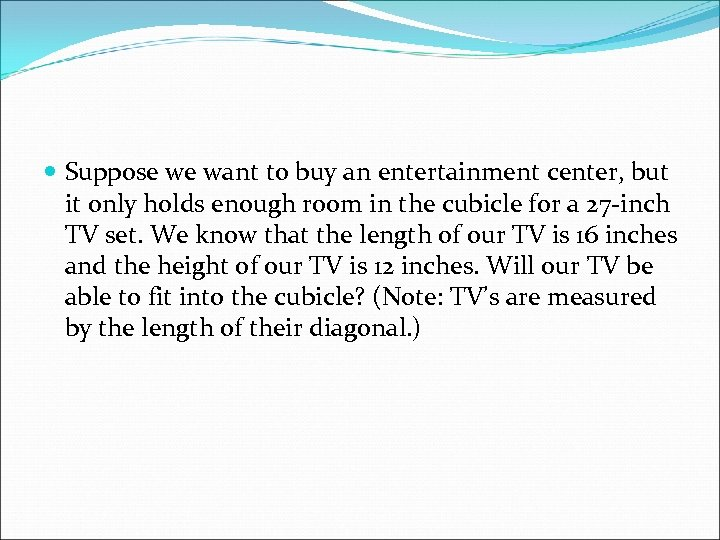Suppose we want to buy an entertainment center, but it only holds enough room in the cubicle for a 27 -inch TV set. We know that the length of our TV is 16 inches and the height of our TV is 12 inches. Will our TV be able to fit into the cubicle? (Note: TV’s are measured by the length of their diagonal. )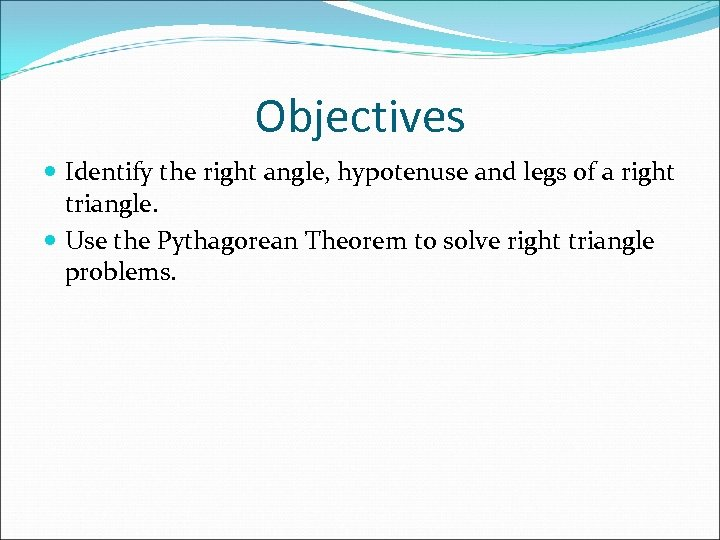Objectives Identify the right angle, hypotenuse and legs of a right triangle. Use the Pythagorean Theorem to solve right triangle problems.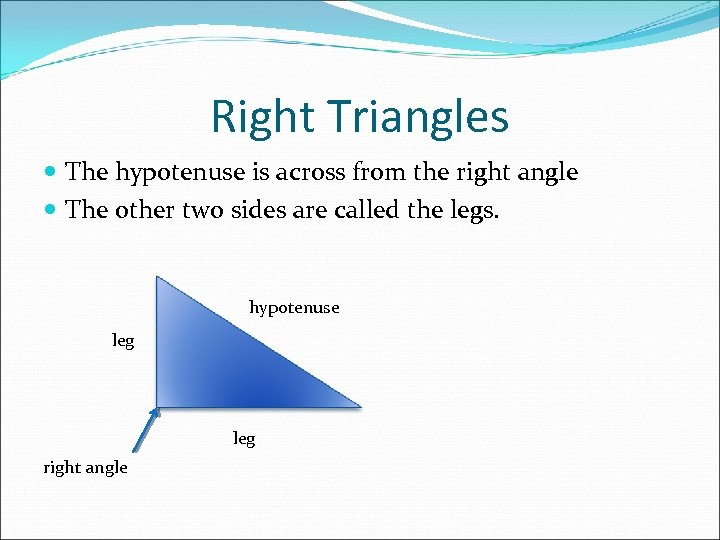Right Triangles The hypotenuse is across from the right angle The other two sides are called the legs. hypotenuse leg right angle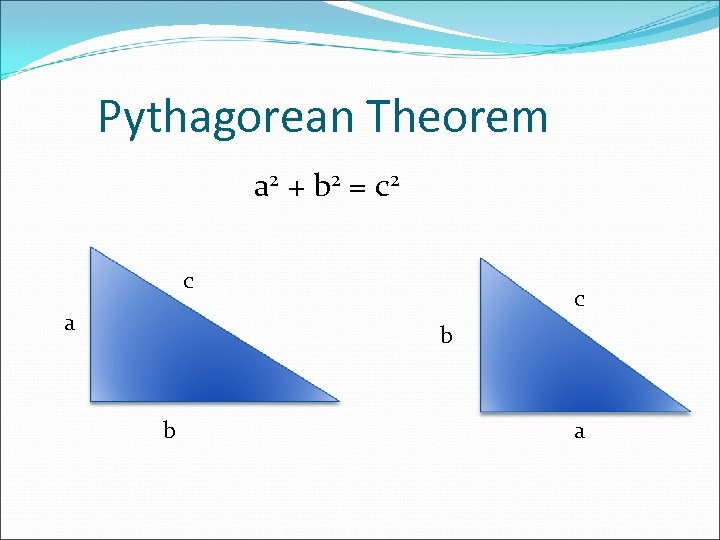Pythagorean Theorem a 2 + b 2 = c 2 c a c b b aNow let’s do some examples!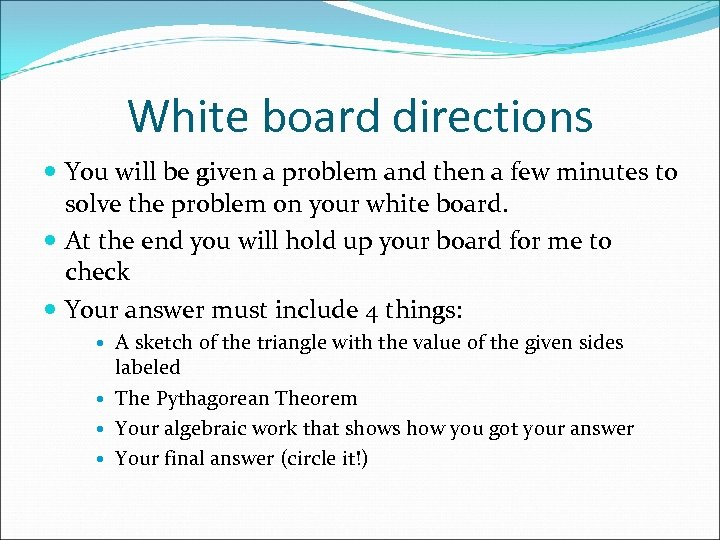White board directions You will be given a problem and then a few minutes to solve the problem on your white board. At the end you will hold up your board for me to check Your answer must include 4 things: A sketch of the triangle with the value of the given sides labeled The Pythagorean Theorem Your algebraic work that shows how you got your answer Your final answer (circle it!)Question #1 A right triangle has a hypotenuse of 15 and a leg of 9. What is the length of the other leg?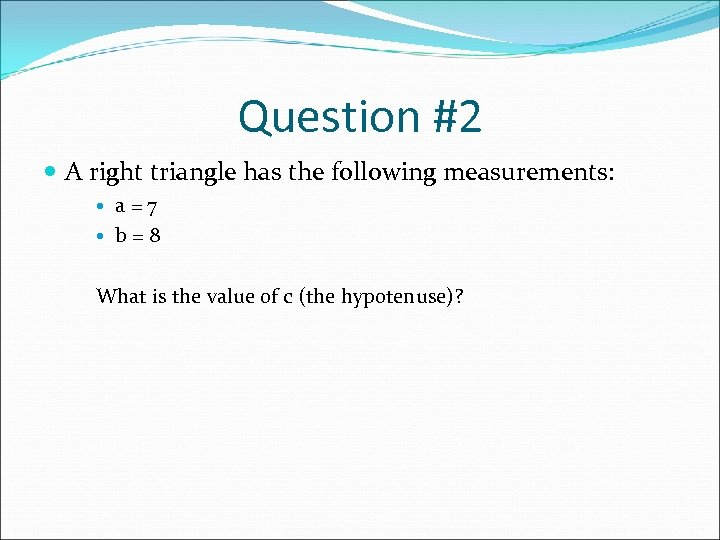Question #2 A right triangle has the following measurements: a=7 b=8 What is the value of c (the hypotenuse)?Question #3 A right triangle has a hypotenuse of √ 50 and a leg of 4. Find the length of the other leg.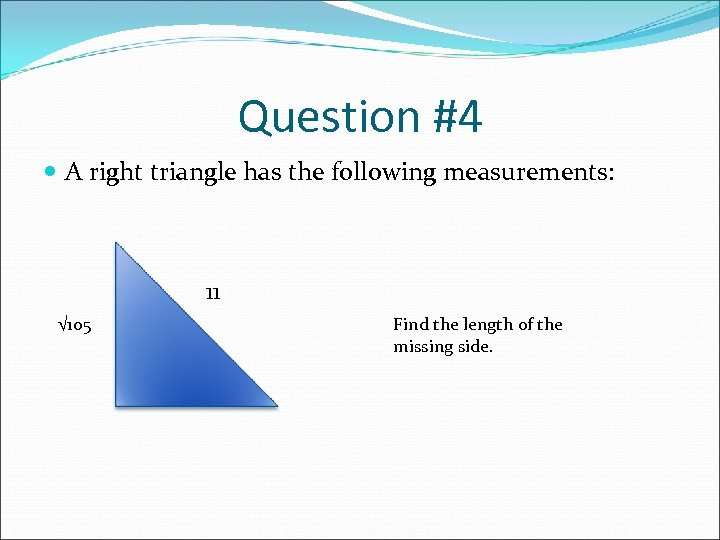Question #4 A right triangle has the following measurements: 11 √ 105 Find the length of the missing side.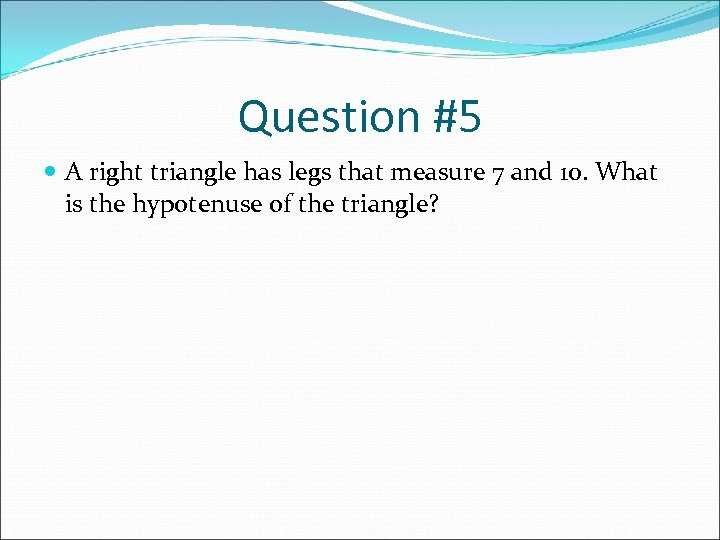Question #5 A right triangle has legs that measure 7 and 10. What is the hypotenuse of the triangle?Objectives Identify the right angle, hypotenuse and legs of a right triangle. Use the Pythagorean Theorem to solve right triangle problems.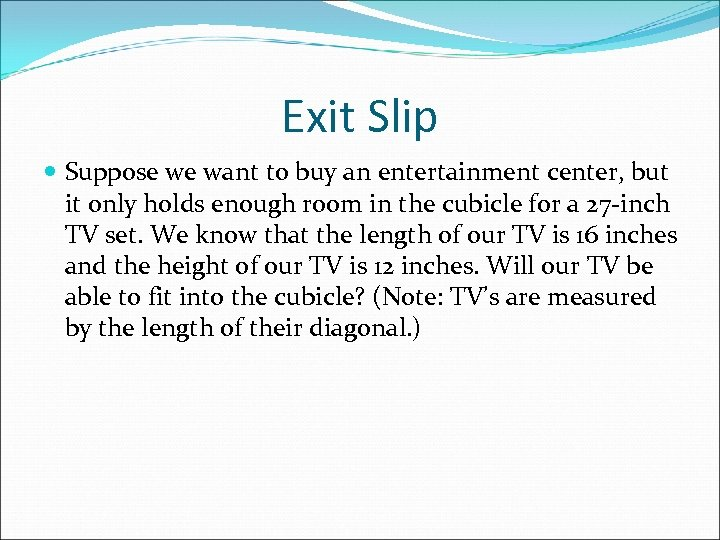Exit Slip Suppose we want to buy an entertainment center, but it only holds enough room in the cubicle for a 27 -inch TV set. We know that the length of our TV is 16 inches and the height of our TV is 12 inches. Will our TV be able to fit into the cubicle? (Note: TV’s are measured by the length of their diagonal. )Don’t forget to do your homework!